Next: Comparison of the DNEB/L-BFGS Up: Results Previous: Comparison of SQVV and   Contents

## Doubly Nudged Elastic Bands

The NEB/QVV approach has previously been systematically tested on systems with around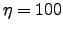degrees of freedom . However, in the majority of cases these test systems could be divided into a core' and a smaller part that actually changes significantly. The number of active degrees of freedom is therefore significantly smaller than the total number in these tests. For example, prototropic tautomerisation of cytosine nucleic acid base (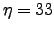) involves motion of one hydrogen atom along a quasi-rectilinear trajectory accompanied by a much smaller distortion of the core.

We have therefore tested the performance of the NEB/SQVV and NEB/L-BFGS schemes for more complicated rearrangements of Lennard-Jones (LJ) clusters to validate the results of Section 2.5.2, and to investigate the stability and performance of both approaches when there are more active degrees of freedom. Most of our test cases involve permutational isomerisation of the LJ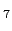, LJ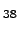and LJ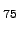clusters.These examples include cases with widely varying separation between the endpoints, integrated path length, number of active degrees of freedom and cooperativity.

Permutational rearrangements are particularly interesting because it is relatively difficult to produce an initial guess for the NEB run. In contrast, linear interpolation between the endpoints was found to provide a useful initial guess for a number of simpler cases . For example, it was successfully used to construct the NEB for rearrangements that involve one or two atoms following approximately rectilinear trajectories, and for migration of a single atom on a surface . For more complex processes an alternative approach adopted in previous work is simply to supply a better initial guess by hand', e.g. construct it from the images with unrelaxed geometries containing no atom overlaps . The detour' algorithm described in previous calculations that employ the ridge method could also be used to avoid atom-crashing' in the initial interpolation .

It has previously been suggested that it is important to eliminate overall rotation and translation (ORT) of each image during the optimisation of an NEB . We have implemented this constraint in the same way as Jónsson et al., by freezing one atom, restricting the motion of a second atom to a plane, and constraining the motion of a third atom to a line by zeroing the appropriate components of the NEB gradient.

We were able to obtain stable convergence in NEB/L-BFGS calculations only for simple rearrangements, which confirms that straightforward L-BFGS optimisation of the NEB is unstable . Figure 2.6 shows the performance of the NEB/SQVV [Figure 2.6 (a)] and NEB/L-BFGS [Figure 2.6 (b)] approaches for one such rearrangement. These calculations were carried out using a 7-image NEB both with (diamonds) and without (stars) removing ORT for isomerisation of an LJcluster (global minimum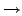second-lowest minimum). The number of iterations,, is proportional to the number of gradient evaluations regardless of the type of minimiser. Hence, from Figure 2.6 we conclude that for this system NEB/L-BFGS is faster than NEB/SQVV by approximately two orders of magnitude. However, removal of ORT leads to instability in the NEB/L-BFGS optimisation: the images do not stay in proximity to the required path for long and instead diverge from it [see inset in Figure 2.6 (b)].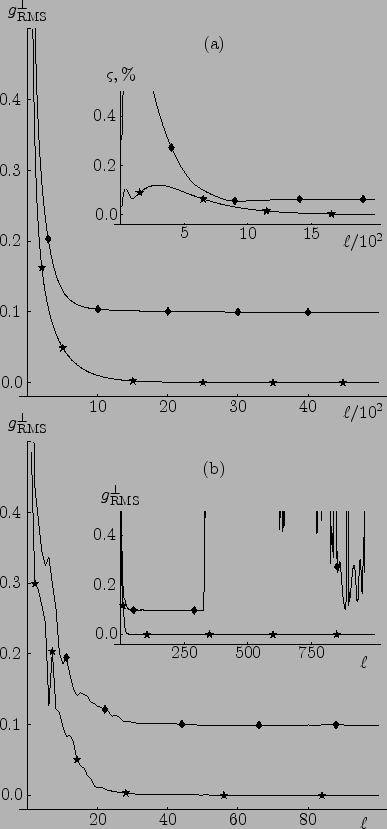By experimentation we have found that the main source of the instabilities is the complete removal of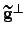. Instead, the inclusion of some portion ofin the NEB gradient, i.e.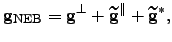(4.39)

where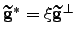, makes the NEB/L-BFGS calculations stable but introduces some additional corner-cutting, as well as an extra parameter,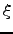. Since we use the transition state candidates from NEB as starting points for further EF calculations the corner-cutting is not a drawback as long as the transition state candidates are good enough. By adjustingin the range of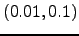we were able to achieve satisfactory performance for the NEB/L-BFGS method in a number of cases. However, an alternative modification, described below, proved to be even more successful.

The drawback of the NEB gradient described by Equation 2.39 stems from the interference of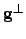and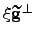, and becomes particularly noticeable when the projection ofonanditself are of comparable magnitude. This problem is analogous to the interference of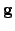and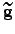in the original elastic band method, which was previously solved by nudging' . We have therefore constructed the gradient of a new doubly' nudged elastic band (DNEB) using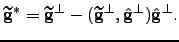(4.40)

In this formulation some corner-cutting may still occur because the images tend to move cooperatively during optimisation; the spring gradient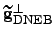acting on one image can still indirectly interfere with the true gradients of its neighbours. In our calculations this drawback was not an issue, since we are not interested in estimating properties of the path directly from its discrete representation. Instead we construct it from steepest-descent paths calculated after converging the transition states tightly using the EF approach. We have found DNEB perfectly adequate for this purpose.

We have implemented DNEB method in our OPTIM program . Equation 2.2 was used to obtain components of both spring gradient and true gradient, Equation 2.4 to calculate pathway tangent, and Equations 2.39-2.40 to evaluate the final DNEB gradient. We note that although we employed the improved tangent from Equation 2.4, we did not use Equation 2.5 to obtain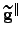. This implementation detail was not clarified in Reference TrygubenkoW04, and we thank Dr. Dominic R. Alfonso for pointing that out.

We have also tested a number of approaches that might be useful if one wants to produce a full pathway involving a number of transition states for a complicated rearrangement in just one NEB run. One of these, for instance, is a gradual removal of the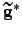component from the NEB gradient once some convergence criterion is achieved. This removal works remarkably well, particularly in situations with high energy initial guesses, which occur frequently if the guessing is fully automated. This adjustment can be thought of as making the band less elastic in the beginning in order to resolve the highest-energy transition state regions first.Next: Comparison of the DNEB/L-BFGS Up: Results Previous: Comparison of SQVV and   Contents
Semen A Trygubenko 2006-04-10